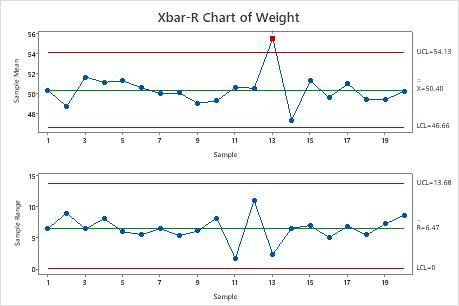# Interpret the key results for Xbar-R Chart

Complete the following steps to interpret an Xbar-R chart. Key output includes the Xbar chart, R chart, and test results.

## Step 1: Examine the R chart to determine whether the process variation is in control

Before you interpret the Xbar chart, examine the R chart to determine whether the process variation is in control. If the R chart is not in control, then the control limits on the Xbar chart are not accurate.

The R chart plots the subgroup ranges. If the subgroup size is constant, then the center line on the R chart is the average of the subgroup ranges. If the subgroup sizes differ, then the value of the center line depends on the subgroup size, because larger subgroups tend to have larger ranges. The control limits on the R chart, which are set at a distance of 3 standard deviations above and below the center line, show the amount of variation that is expected in the subgroup ranges.

Red points indicate subgroups that fail at least one of the tests for special causes and are not in control. If the same point fails multiple tests, then the point is labeled with the lowest test number to avoid cluttering the graph. If the chart shows out-of-control points, investigate those points.

Out-of-control points can influence the estimates of process parameters and prevent control limits from truly representing your process. If out-of-control points are due to special causes, then consider omitting these points from the calculations. For more information, go to Specify how to estimate the parameters for Xbar-R Chart.No points are out of control on the R chart (the bottom chart).

## Step 2: Examine the Xbar chart to determine whether the process mean is in control

The Xbar chart plots the average of the measurements within each subgroup. The center line is the average of all subgroup averages. The control limits on the Xbar chart, which are set at a distance of 3 standard deviations above and below the center line, show the amount of variation that is expected in the subgroup averages.

Red points indicate subgroups that fail at least one of the tests for special causes and are not in control. If the same point fails multiple tests, then the point is labeled with the lowest test number to avoid cluttering the graph. If the chart shows out-of-control points, investigate those points.

Out-of-control points can influence the estimates of process parameters and prevent control limits from truly representing your process. If out-of-control points are due to special causes, then consider omitting these points from the calculations. For more information, go to Specify how to estimate the parameters for Xbar-R Chart.In these results, the R chart is stable, so it is appropriate to interpret the Xbar chart. Look at the top chart. One point is out of control on the Xbar chart. Subgroup 13 fails test 1. When you hold the pointer over a red point, you can see more information about the subgroup.

## Step 3: Identify which points failed each test

Investigate any subgroups that fail the tests for special causes. By default, Minitab conducts only Test 1, which detects points that fall outside of the control limits. However, if you conduct additional tests, then points can fail multiple tests. The output shows exactly which points failed each test, as shown here.

TEST 1. One point more than 3.00 standard deviations from center line. Test Failed at points: 12 TEST 2. 9 points in a row on same side of center line. Test Failed at points: 12 * WARNING * If graph is updated with new data, the results above may no longer be correct.
###### Note

When you use several tests at the same time, the sensitivity of the chart increases. However, the false alarm rate also increases, which can make you react to the test results unnecessarily.

For more information on each of the tests and when to use them, go to Using tests for special causes in control charts.

By using this site you agree to the use of cookies for analytics and personalized content.  Read our policy